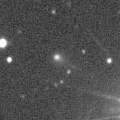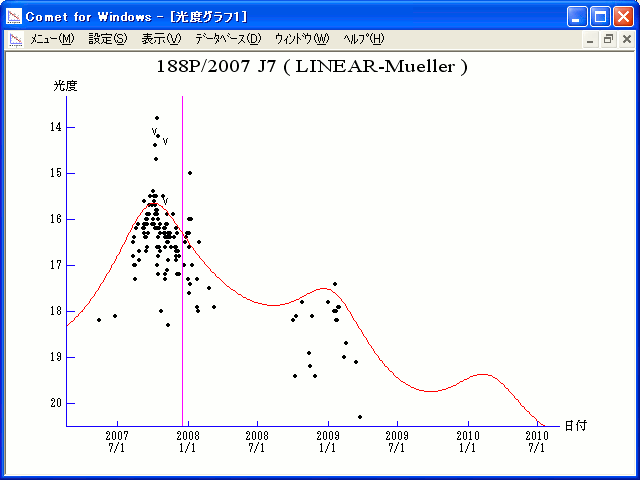# \$B%j%K%"(B-\$B%_%e%i!

188P/LINEAR-Mueller (2007)###\$B%W%m%U%#!<%k(B

 \$BId9f(B 188P/2007 J7 \$B8!=PF|(B 2007\$BG/(B5\$B7n(B13\$BF|!"(B6\$B7n(B26\$BF|(B \$B8!=P8wEY(B 18.2\$BEy!"(B18.1\$BEy(B \$B8!=P R. H. McNaught (Siding Spring), J. V. Scotti (Kitt Peak)

###\$B###\$B50F;MWAG(B

```                    Epoch = 2007 Dec.  6.0 TT
T = 2007 Dec. 16.1419 TT         Peri. =  26.4358
e = 0.415879                     Node  = 359.1499  2000.0
q = 2.552135 AU                  Incl. =  10.5462
a =  4.369188 AU    n = 0.1079202    P =   9.133 years
```

###\$B@1?^(B###\$B8wEYJQ2=(B

```        m1 = 12.0 + 5 log\$B&\$(B + 6.3 log r
```##### \$B50F;MWAG\$O!"(B IAUC 8853 \$B\$K7G:\\$5\$l\$?\$b\$N\$G\$9!#(B \$B@1?^\$O(B StellaNavigator Ver.2.0 for Windows (\$B%"%9%H%m%"!<%D(B \$BJTCx(B / \$B%"%9%-!<=PHG6I4)(B) \$B\$G:n@.\$7\$?\$b\$N\$G\$9!#(B \$B8wEY%0%i%U\$O(BComet for Windows\$B\$G:n@.\$7\$?\$b\$N\$G\$9!#(B